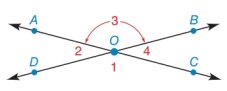Chapter 1.7, Problem 19E### Elementary Geometry for College St...

6th Edition
Daniel C. Alexander + 1 other
ISBN: 9781285195698

#### Solutions

Chapter
Section### Elementary Geometry for College St...

6th Edition
Daniel C. Alexander + 1 other
ISBN: 9781285195698
Textbook Problem
1 views

# In Exercises 19 to 26, use the drawing in which A C ↔ intersects D B ↔ at point O.If m ∠ 1 = 125 ° , find m ∠ 2 , m ∠ 3 and m ∠ 4 .To determine

To find:

The value of m2, m3 and m4.

Explanation

a. If two lines intersect, then measure of vertical angles formed is equal.

b. The sum of angles formed on a straight line is supplementary.

Given:

The given angle is m1=125°.

The given figure is,

Calculation:

From the given figure it can observed that 1 and 3 are vertical angles. The measure of vertical angles is equal.

m3=m1=125°

The angle 2 is supplementary to 3 as DB is a straight line.

m2+m3=180°

Substitute 125° for m3 in above equation.

### Still sussing out bartleby?

Check out a sample textbook solution.

See a sample solution

#### The Solution to Your Study Problems

Bartleby provides explanations to thousands of textbook problems written by our experts, many with advanced degrees!

Get Started

#### 38. For Are and defined? What size is each product? Can =? Explain.

Mathematical Applications for the Management, Life, and Social Sciences

#### Proof Prove Theorem 13.14.

Calculus: Early Transcendental Functions (MindTap Course List)

#### f(x) = x3 2x2 + x 1 has a local maximum at: a) 0 b) 1 c) 13 d) f has no local maxima

Study Guide for Stewart's Single Variable Calculus: Early Transcendentals, 8th